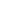# The simple interest on a sum of money is 1/9 th of the principal and the number of year is equal to the rate percent per annum. The rate of interest per annum is:

a) 2 %
b) 7 %
c) 9%
d) 10/3 %

 Ans: d. Solution Let Principal = x SI = x/9 R = T;R =10/3
Share :
+
Next
« Prev
Prev
Next »